#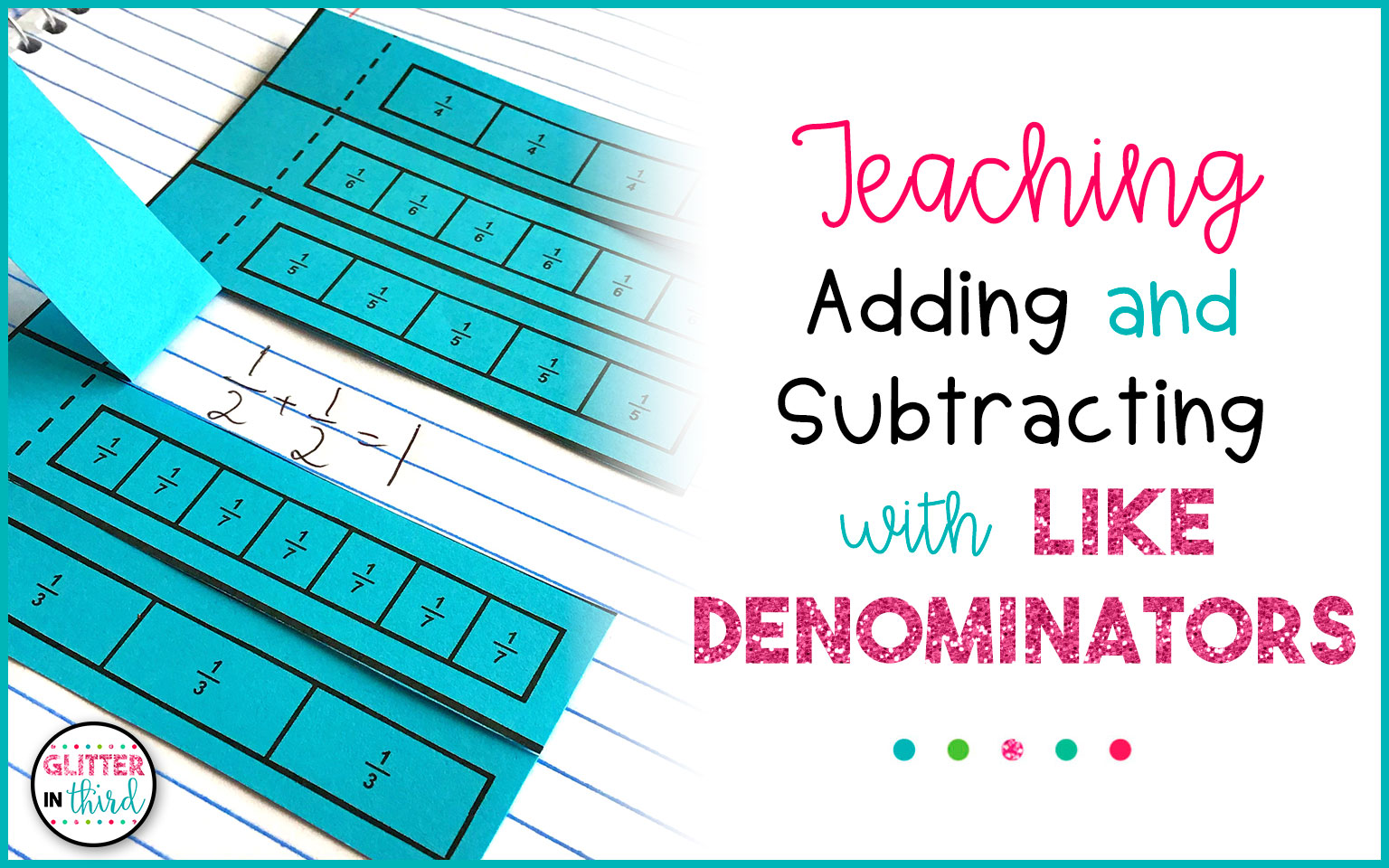Looking for adding and subtracting fractions with like denominators activities?

Just when your students have gotten comfortable with fractions… Boom!

You throw a whole new skill at them and it feels like they’re learning a different language.Adding and subtracting fractions with like denominators is a relatively simple skill to learn, but there are a lot of aspects that play into the lesson besides just adding and subtracting.

By this age your students have a good foundation of basic addition and subtraction, so finding resources and activities to challenge these skills is important!

While you may be thinking working with fractions is challenging enough, the resources I’m going to share with you will help your students work with like denominators in multiple different ways and combat their critical thinking skills.

I’ve also included some tips for helping students simplify their fractions after adding or subtracting, but I won’t get ahead of myself just yet!## Adding and subtracting fractions activities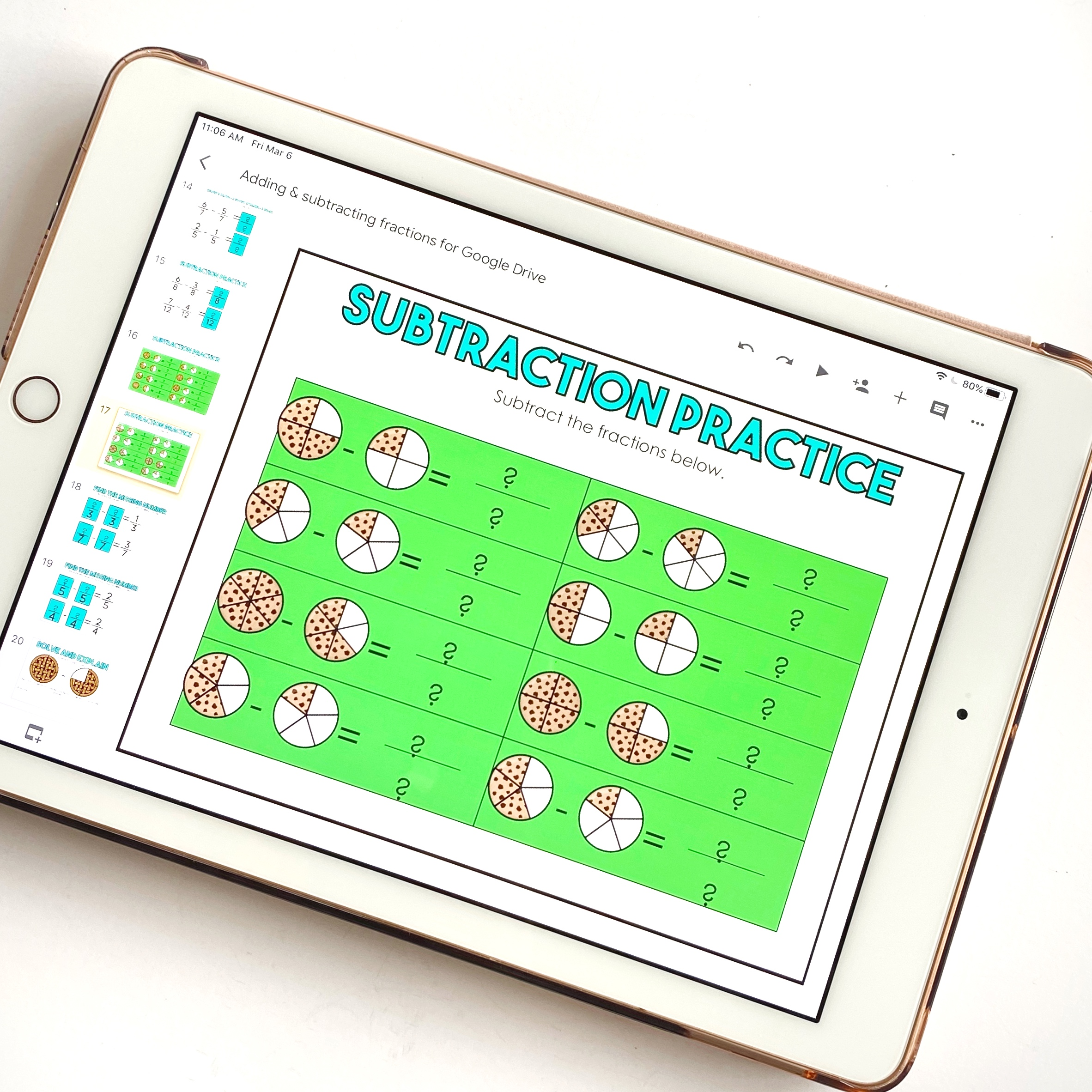One of the best ways you can help your students understand adding and subtracting fractions is presenting it through both numbers and models.

Some kids have no problems learning with just numbers, while others learn best through physical representation – this resource includes both!

Included in this Google Classroom resource is a plethora of short-answer activities that address both adding and subtracting numerical fractions, and visual fractions in the form of pizzas, cupcakes, and cookies.

You’ll find anywhere from your basic equations such as ⅗ + ⅕ to more complex questions that require your students to find missing addends or to create given amounts by adding or subtracting fraction portions.

For example, what’s a possible equation that could make 7/10?

Your students will be able to work with fraction tiles to represent 3/10 + 4/10 = 7/10,  5/10 + 2/10 = 7/10, etc.

There are also word problems included that require your students to show a step-by-step method for how they solved the problem.

Just another way to build a deeper understanding of the skill and allow you to see their thinking and solving process.

To grab this Google Classroom resource, you can find it here

### Interactive Notebook for Adding and Subtracting Fractions with Like Denominators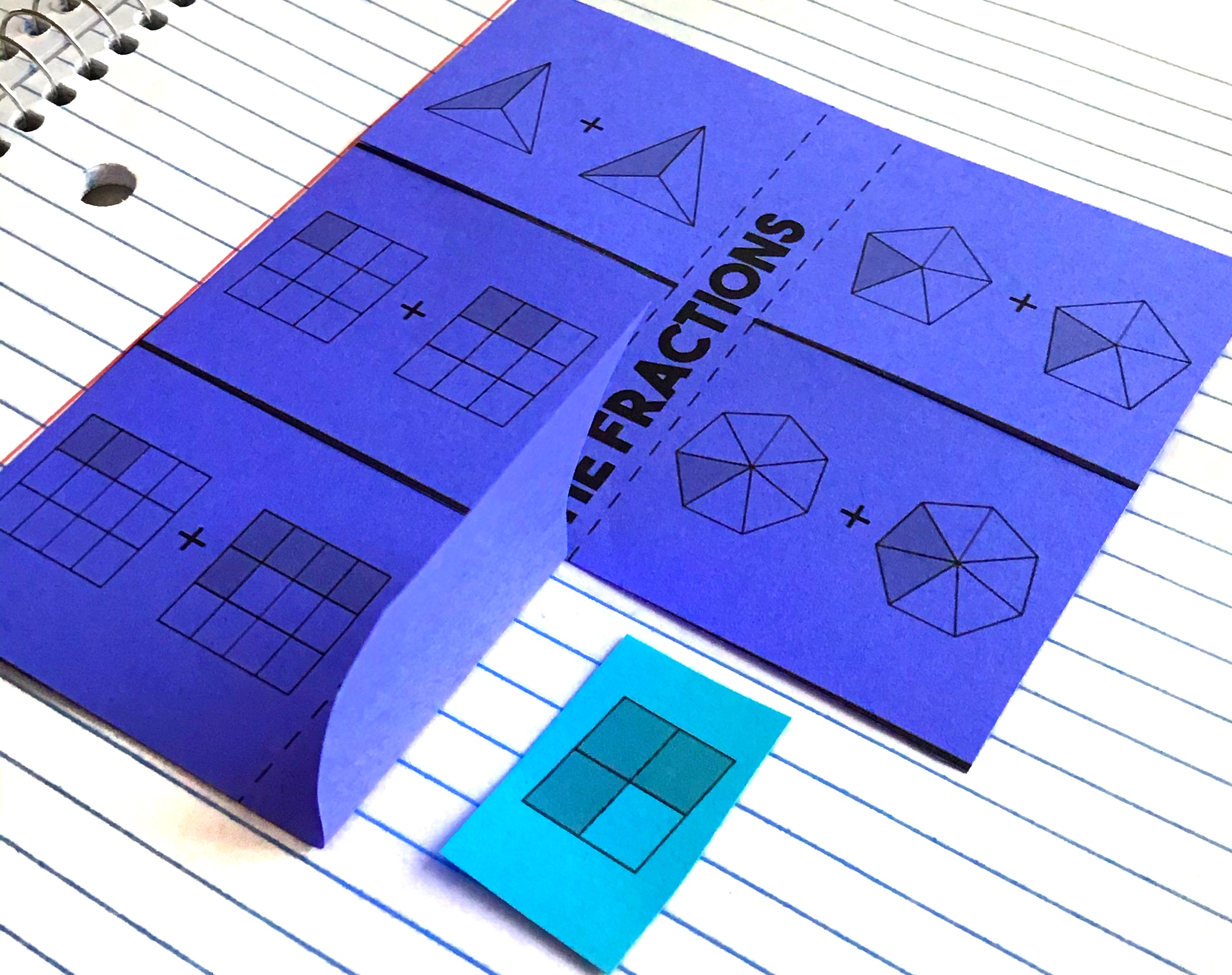This interactive notebook is another great option for going beyond the basic addition and subtraction problems.

Like the Google Classroom resource, but with all different questions and content, there are activities that require filling in missing fractions to addition or subtraction problems, as well as questions that contain mistakes for the students to find and explain what is required to correct the mistake.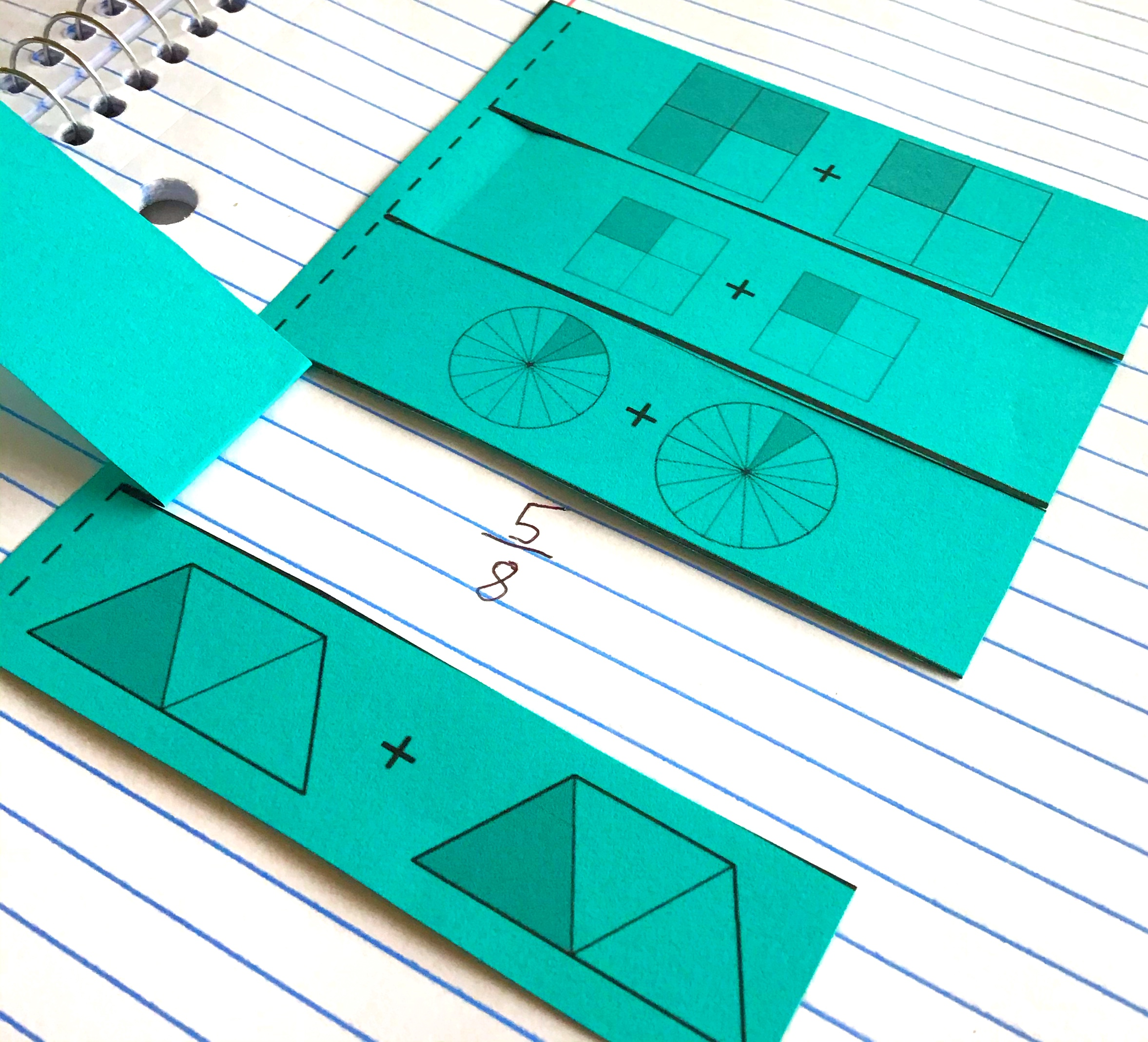Questions are in both numerical form and model form to support all students’ preferred learning types, as well as word problems to extend their learning even more.

There are 3 levels of differentiation to allow for any accommodation.

This notebook can also be converted into an interactive PDF if you prefer digital over paper!

You can take a closer look at this resource here!### Simplifying Fractions

I honestly think the most difficult part of adding and subtracting fractions with like denominators isn’t the actual addition or subtraction part, but simplifying the answer.

Before your students can confidently start simplifying their answers, they’ll have to do some groundwork with prime factorization.

I’ll do some debriefing on prime factorization if you’re needing a quick refresh!The first step in simplifying is to find the greatest common factor between the numerator and the denominator.You can do this by completing the prime factorization for each number.

We can practice with 9/12.

The prime factorization for 9 = 3 x 3.

3 can’t be broken down anymore, so we have to leave it as is.

The prime factorization for 12 = 4 x 3.

4 can be broken down again to 2 x 2.

The greatest common factor between 9 and 12 is 3.

9 divided by 3 is 3, and 12 divided by 3 is 4. So that means 9/12 simplified is ¾.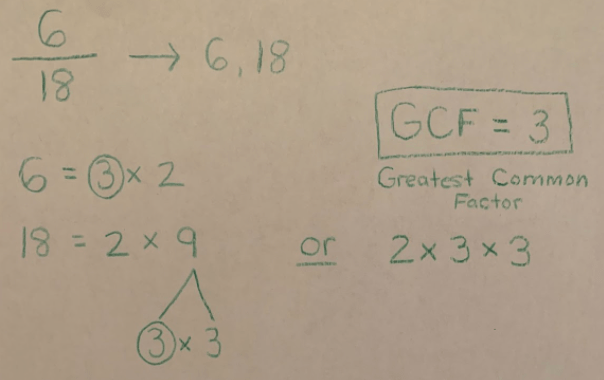I have included a couple more examples below!

I like to add in these examples because they either require another step, or they’re solved differently!

6 divided by 3 is 2.

18 divided by 3 is 6.

9 times out of 10 your students will walk away writing 2/6 as their simplified fraction.Help them see they have one more prime factorization to solve since both 2 and 6 are divisible by 2!

Therefore making the actual simplified answer ⅓.

5 is also a great prime number to work with since 5 alone is prime, but other numbers that contain 5 are not prime.

5 divided by 5 is 1. 15 divided by 5 is 3, making this simplified fraction also ⅓.

### Fraction Bars or Strips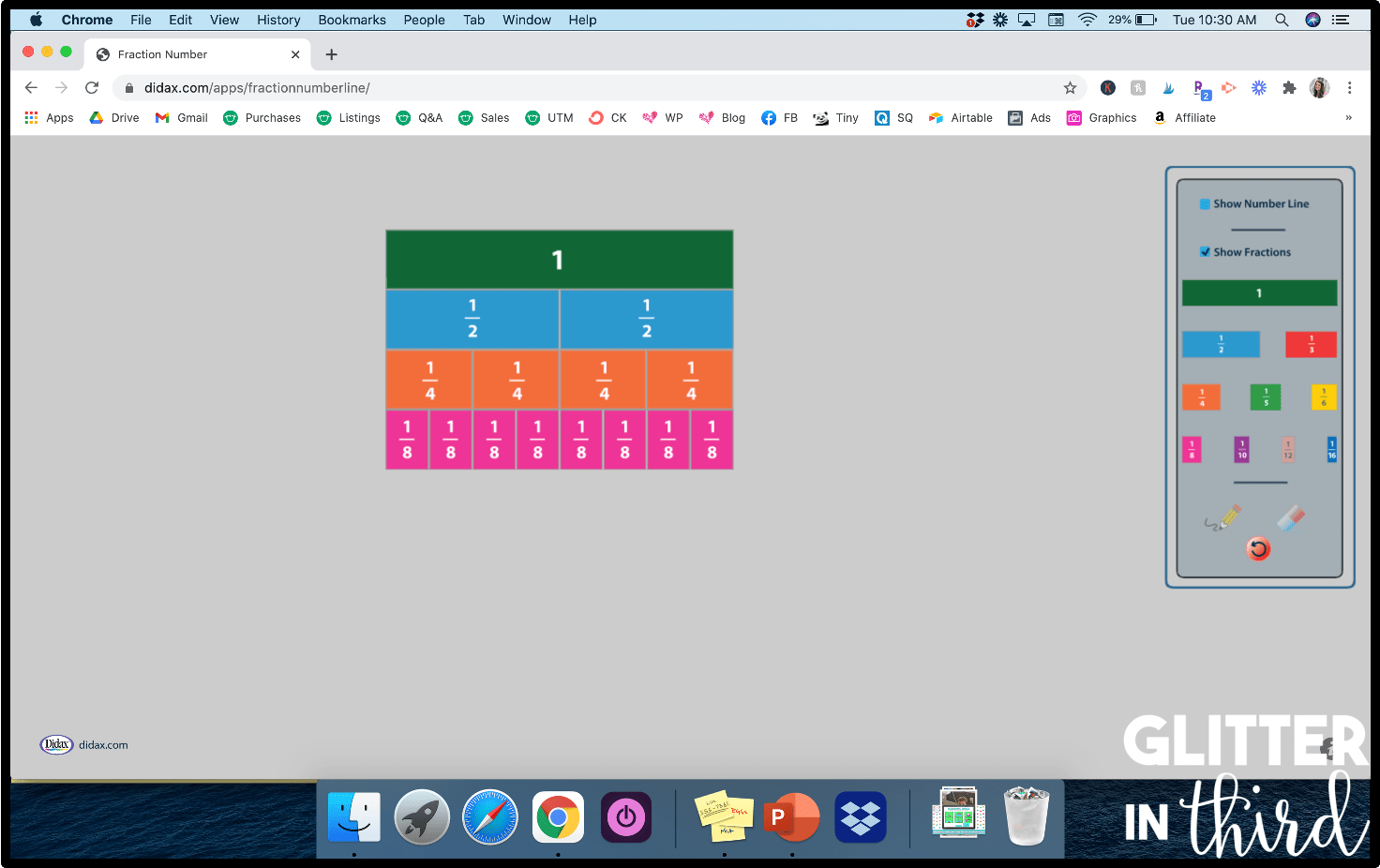If you’re looking for one of the most basic ways to teach adding and subtracting fractions with like denominators, that also focuses on simplifying, fraction bars or strips are SO helpful to provide a visual!

These fraction bars show multiple ways to add up to 1.

It can also show that 4/8 is equal to 2/4, as is ½.

If your students are struggling with understanding what it means to “simplify,” I highly recommend showing them these kinds of displays.

Visuals can totally clear up the confusion!

There are multiple digital fraction bars and strips that you can use for free – Toy Theater, Didax, and Room Recess are some online resources just to name a few!

### Music

Another form of learning that your students may connect with is through music and song.

To us they may seem cheesy.To your students they may be lifesavers!

You can listen to the simplest form song by Numberock here!

I also think this simplifying fractions rap is super fun.

Not only is it a catchy beat that your students remember, but also incorporates a pizza visual and numbers, too. You can preview it here!

## Want an equivalent fractions freebie?Enter your deets below to get an equivalent fractions color-by-number activity sent straight to your inbox!

## Looking for more fraction blog posts?

I have a number of other fraction blog posts for you to check out!

The post Adding and Subtracting Fractions with Like Denominators appeared first on Glitter in Third.